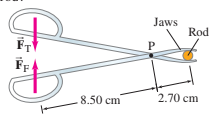# Problema 1 (6)

Enllaç a codi Mathjax d'equació de segon grau

The solution of the problem step by is as follows

Expand the following: \begin{align} ΣF_y=0 ->&\cssId{Step1}{F_a × D_1 - F_b × D_2 =0}\\[3px] &\cssId{Step2}{F_a × D_1 = F_b × D_2}\\[3px] &\cssId{Step3}{\frac{F_a × D_1}{D_2} = F_b}\\[3px] \end{align}
1. The figure shows a pair of forceps used to hold a thin plastic rod firmly. If the thumb and finger each squeeze with a force what force do the forceps jaws exert on the plastic rod?Please enter the following data to calculate the previous equation

 Enter Force for the forceps: N Enter distance D1: cm Enter distance D2: cm Equation Force rod result is: Comments about initial values Comments about results